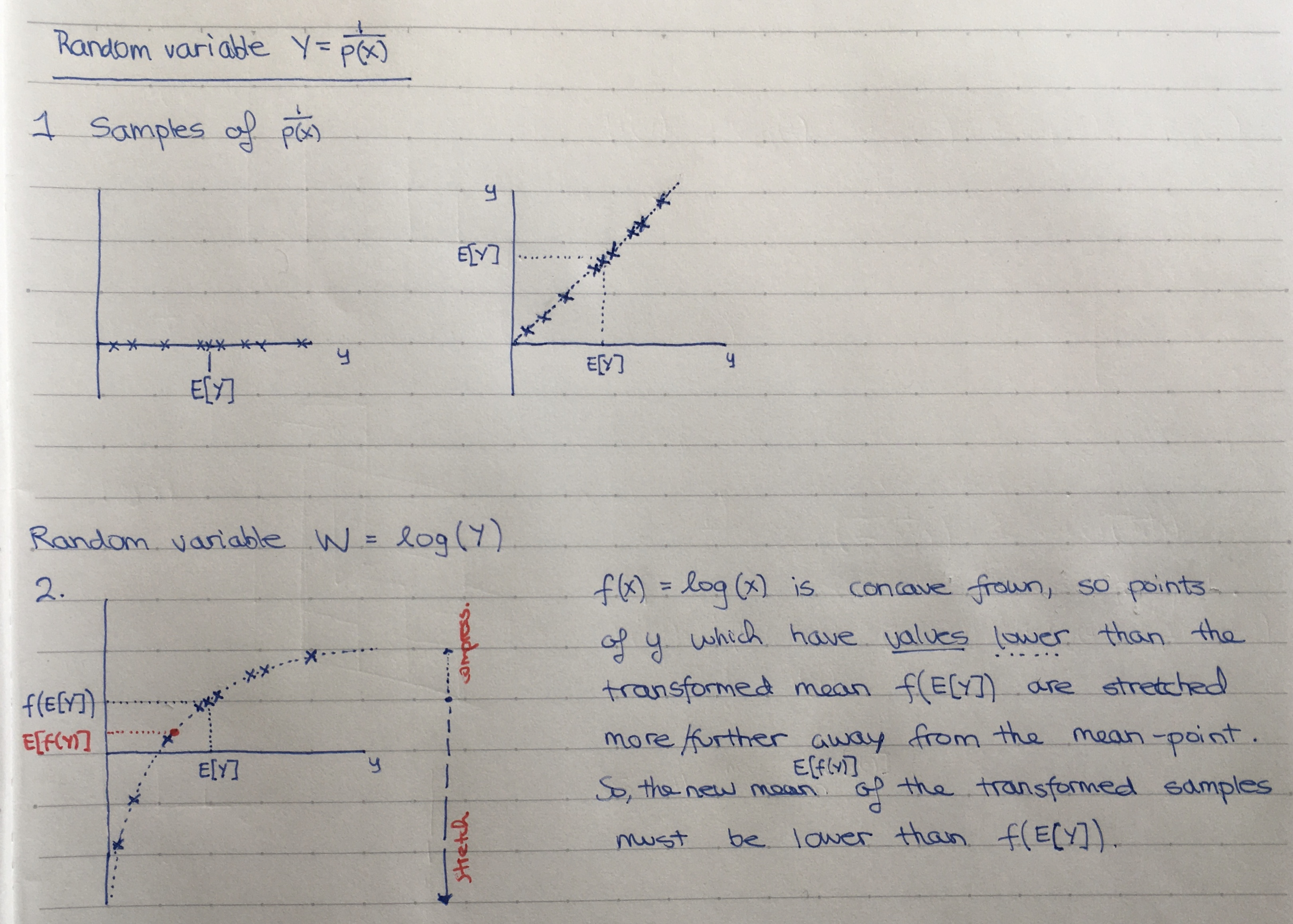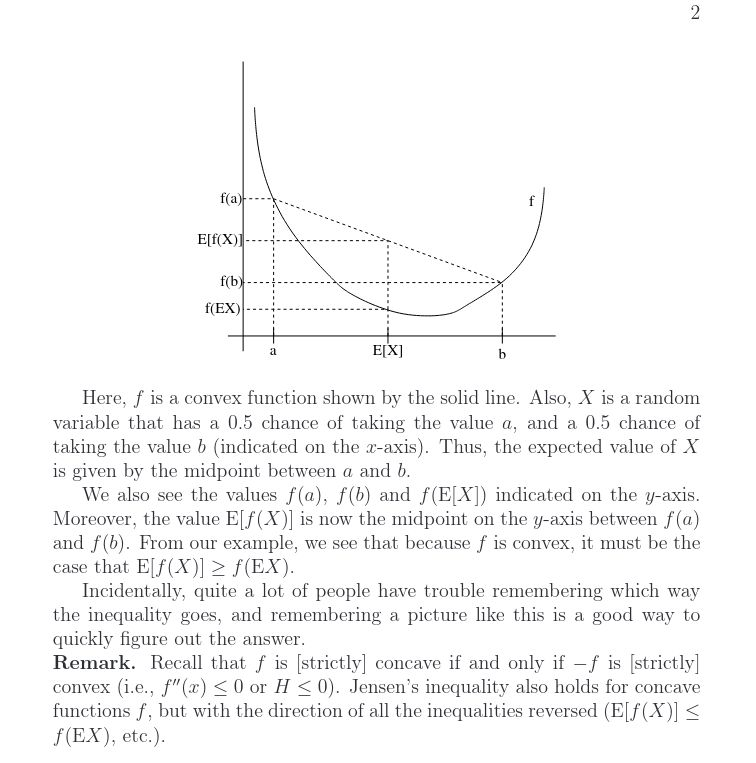Show Question
Jensen's inequality

If $$f$$ is a convex (smile) function and $$X$$ is a random variable then:

$E[f(X)] \ge f(E[X])$
If $$f$$ is strictly convex and $$E[f(X)] = f(E[X])$$, then the random variable $$X$$ is a constant.

Intuition:Proof:

#### Intuition #2

The following is taken from Andrew Ng's notes for CS229:TODO

## Example

Q1: Three squares

Three squares have average area $$\bar{A} = 100 m^2$$. The average of the lengths of their sides is $$\bar{l} = 10 m$$. What can be said about the size of the largest of the three squares?

A1:
Let $$x$$ be the length of the side of a square, and let the probability of $$x$$ be $$\frac{1}{3}, \frac{1}{3}, \frac{1}{3}$$ over the three lengths, $$l_1, l_2, l_3$$. Then the information that we have is:

* $$E[X] = 10$$
* $$E[f(X)] = 100 \text{, where } f(x) = x^2$$

$$f$$ is a strictly convex function and the equality $$E[f(X)] = f(E[X])$$ holds, so by Jensen's equality, $$x$$ must be a constant and all three lengths must be equal. So the area of the largest square (and all squares) is $$100 m^2$$.